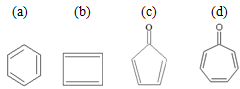# Solved Examples on Hydrocarbons

Question:1 Which of the following molecules, in pure form, is (are) unstable at room temperature? (IIT JEE 2013)Solution:  b and c are anti-aromatic molecules as these contain 4n i.e. 8 π electrons in ring and hence, these are highly unstable.Anti aromatic compounds are those for which number of ring π electrons is equal to 4n (i.e. 4n = 4 or n=1).Question :2. In allene (C3H4), the type(s) of hybridization of the carbon atoms is (are)  (IIT JEE-2011)

a. sp & sp3
b. sp and sp2
c. only sp2
d. sp2 and sp3

Solution: Allene are the molecules in which at least one C has double bonds with each of the adjacent carbon atoms..Structure of the allene C3H4 isThe central carbon forms two sigma and two pi bonds with the two adjacent carbon atoms, so the central carbon is sp and the two terminal carbons are sp2 hybridised.
Hence, the correct option is B.

Question: 3 The number of optically active products obtained from the complete ozonolysis of the given compound is(IIT JEE-2011)

a. 0

b. 1

c. 2

d. 4

Solution: Ozonolysis reactionOzonolysis of one molecule of the given compound results in the formation of two products CH3CHO & OHCHCH(CH3)CHO, two molecules each. None of these is optically active.

For being optically active any molecule must contain a chiral carbon atom (i.e. the carbon atom which is attached to the four different groups). None of the products formed has the chiral carbon and thus these all are optically inactive.

Hence, the correct option is A.